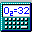##Overview of Features

Click the contents button to see a list of available help topics.
(may need to click the Show button at the upper left)

### Tools

Mole/Mass/Dilution Converter for easily interconverting moles and mass, for computing molarity or concentration given an amount and volume, and for performing dilution calculations.

Formula Finder for finding potential empirical formulas for a given molecular weight or for a given set of percent composition data. Includes a Smart Hydrogen feature to limit the number of hydrogen atoms in found compounds to a reasonable number.

Amino Acid Notation Converter to convert between 3 letter and 1 letter abbreviations for amino acids (converts both ways).

Peptide Sequence Fragmentation Modelling module to predict the ion masses expected from fragmentation of a peptide in the collision cell of a mass spectrometer. Actual experimental data may also be matched to a theoretical peptide sequence.

Capillary Calculations module for performing typical flow, mass-rate, and band broadening calculations in open and packed capillary columns, commonly used in capillary liquid chromatography

Isotopic Distribution module for predicting the masses and abundances of the isotopes for a given formula, useful for comparison to observed mass spectrometry results

Built-in mathematical calculator that follows mathematical precedence rules.

### Features

Multi Line Display Display of up to 20 formulas with their molecular weights simultaneously (dependent upon screen resolution).

Percent Composition Elemental percent composition of each of the entered formulas.

Parentheses are allowed
For example, CH3(CH2)3CH3 is equivalent to CH3CH2CH2CH2CH3
Can also handle decimal numbers following parentheses.
For example, (Fe2O3).5 is equivalent to FeO1.5
Hydrates or other appended compounds are allowed.
For example, FeCl3-6H2O or FeCl3-2.5H2O
Can also begin a formula with a number.
For example, 4FeCl3-6H2O is equivalent to (FeCl3)4-6H2O
User-definable abbreviations Default abbreviations are included for common parts of compounds, including amino acids. See full list.
For example, PhCl = C6H5Cl and
HOAc = CH3COOH.

Smart Case Conversion The program will automatically convert lowercase letters to uppercase where appropriate for ease of entering a formula. Exact case matching and non-conversion are also available.

Isotopes are recognized using the following notation:
^13C is Carbon-13
C6H5^18OH is heavy-oxygen (Oxygen-18) labeled phenol
^78.918Br is Bromine 79 with a specific isotopic mass
Convert a formula to its empirical formula
For example, HOC6H4Cl would become C6H5ClO
Feature of weighting parts of a compound relative to the other parts. For example:
[.2Na]Cl would have a weight of 0.2*22.989768+35.4527=40.0507

NaCl-.5H2O would have a weight of 22.989768+35.4527+0.5*(2*1.00794+15.9994)=67.4501

Feature of Element Subtraction to return the mass of a formula, minus one or more elements. For example:
C6H5Cl>HCl means C6H5Cl minus HCl, having a mass of 76.0959 Da (average mass), equivalent to C6H4

The overall Charge on a compound can be estimated. The program sums up the default charges for each element and for each abbreviation to give the total charge for a formula. Hydrogens are treated as +1 when preceded by a halogen and -1 when preceded by other elements (including carbon and all metals).

Percent Solver mode for finding the value of "x" in a compound that satisfies user-specified percent composition requirements.

International (foreign language) text phrases may be loaded for all labels and messages in the program. Current languages included are Spanish, German, Portuguese, and Russian. You can make your own language-specific caption file by simply editing a text file.

Edit and save abbreviations while program is running.

Edit and save elemental values while program is running. Further, default elemental weights can be set for the elements' average atomic weights, isotopic weight of the most common isotope, or nominal integer weight of the most common isotope.

Accuracy of the final digit of the molecular wt. and percent composition.

Capability of saving options as defaults and automatic loading of the saved options upon program start.

Capability of saving values and formulas as defaults and automatic loading of the saved values upon program start.

Ability to autosave values, formulas, and options on program exit.

Easily Cut, Copy, and Paste information between the Molecular Weight Calculator and other Windows applications.

Ability to expand abbreviations into their elemental compositions.

Ability to convert a formula to its empirical formula.

Ability to process and compute molecular weights for compounds listed in an input text file. Can also run the Formula Finder in batch mode using a text file. Specify the file by choosing calculate weights from file menu or using the /F:filename switch at command line.

Capability of printing results.

Extensive Help (including context sensitive tool tips) and Error Checking